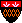Introduction to Automata Theory, Languages, and Computation: Errata for the First, Second, and Third Printings
List of Errata for the First Through Fourth Printings.

In addition, the following were not corrected in time for the third printing, but were corrected for the fourth printing:

p. 18, l. 21: Capitalize "we" in "we must be careful..." (Thanks to Sebastian Hick).

p. 21, l. 4: "right" -> "left" (Thanks to Sebastian Hick).

p. 25, l. 17: The reference to Eq. (1.10) should be to Eq. (1.11) (Thanks to Sebastian Hick).

p. 35, l. 7: "by" -> "be" (Thanks to Sebastian Hick).

p. 42, Fig. 2.2(b): Period after "ship" should be a comma (Thanks to Sebastian Hick).

p. 79, l. 9: "epsilon-DFA" -> "epsilon-NFA" (Thanks to Sebastian Hick).

p. 85, l. -9: "is" before "the set..." (Thanks to Sebastian Hick).

p. 97, l. 1 of text: P_j -> P_i (Thanks to Sebastian Hick).

p. 118, l. 13: The w after "To see why, if" should be w_i (Thanks to Sebastian Hick).

p. 120, l. 4: "than" -> "then" (Thanks to Kirk Bulis).

p. 147, Exercise 4.2.7, l. 1: The last symbol for x should be b_n, not b_m (Thanks to Tapani Lindgren).

p. 196, l. 8: Delete "the" (Thanks to Ben Pfaff).

p. 216, l. 2: "Interion" -> "Interior" (Thanks to Cheryl Dugas).

p. 222, l. 4: "chose" -> "choose" (Thanks to Jennifer Johnson).

p. 233, 4 lines below Fig. 6.5: "than" -> "then" (Thanks to Yingge Wang).

p. 234, l. 11: eliminate one ",epsilon" in (r,epsilon,epsilon) (Thanks to Cheryl Dugas).

p. 276, l. -2: quotes should appear after 'game' rather than 'follows' (Thanks to Guray Alsac).

p. 290, Eq. (7.1): The term Gamma for the stack alphabet is missing (Thanks to Chittaranjan Tripathy).

p. 303, Exercise 7.4.5, l. 1: "so can" -> "to" (Thanks to Christian Lemburg).

p. 316 last line of box: "is" needed before first "undecidable" (Thanks to Christian Lemburg).

p. 321, l. 19: "repeatedly" -> "enters a loop in which it" (Thanks to Christian Lemburg).

p. 355, l. -6: "we" -> "be" (Thanks to Jacinth H.T. Wu).

p. 366, reference 3: The correct capitalization and diacritical marks are: `\"Uber formal unentscheidbare S\"atze der Principia Mathematica und verwandter Systeme´´, Monatshefte f\"ur Mathematik und Physik, Monatshefte für Mathematik und Physik" (Thanks to Jörg Bredno and Christian Lemburg).

p. 379, l. 2: "A efficient" -> "An efficient" (Thanks to Chittaranjan Tripathy).

p. 396, l. 7: the final pair should be (\$,*\$) (Thanks to Zeki Bayram).

p. 400, Fig. 9.15, Rule (3), lines 6-7: The # at the end of List B for the transitions delta(q_2,0) should not be there (Thanks to Ed Luke).

p. 415, l. 15: "that" -> "than" (Thanks to Christian Lemburg).

p. 422, beginning of Section 10.1.6: We do not need to say twice that L is in NP (Thanks to Mustafa Sait-Ametov).

p. 423, l. -10" "in" -> "it" (Thanks to Christian Lemburg).

p. 432, bottom and 433, top: There is a case missing from the design of B_ij. If the state of alpha_i is adjacent to position j, then neither A_{ij} nor B_ij is true as stated. Thus, we have to add terms that make B_ij true in that case. A succinct form for B_ij is:

```     (y_{i,j-1,q1} OR ... OR y_{i,j-1,qm}) OR /* state to left */
(y_{i,j+1,q1} OR ... OR y_{i,j+1,qm}) OR /* state to right */
((y_{i,j,Z1} OR ... OR y_{i,j,Zr}) AND   /* state at least 2 positions away
((y_{i,j,Z1} AND y_{i+1,j,Z1}) OR ... OR (y_{i,j,Zr} AND y_{i+1,j,Zr})))
/* and symbol doesn't change */
```

(Thanks to Zeki Bayram).

p. 433, l. -3: A_ij should be B_ij (Thanks to Christian Lemburg).

p. 435, Ex. 10.2.2, l. 3: quotes needed after j. (Thanks to Mustafa Sait-Ametov).

p. 445, item 1, l. 1: no capital for "Introduce" (Thanks to Christian Lemburg).

p. 452, l. -16, -15: The edge-cover problem is really polynomial-time (Thanks to Christian Lemburg).

p. 458, l. -2: Capitalize "Hamilton" (Thanks to Mustafa Sait-Ametov).

p. 465, l. -18: "time limit" needs quotation marks around it (Thanks to Christian Lemburg).

p. 475, l. 6 of proof of Theprem 11.4: right parenthesis missing at end of exponent on c (Thanks to Chittaranjan Tripathy).

p. 495, l. -11, "and accepting" -> "an accepting" (Thanks to Chittaranjan Tripathy).

Also, thanks to Darren Brown, David Brumley, Michael Dautermann, Gabor Hardy, Rakesh Kumar, Troy Landers, Terry Lewis, Heather Mahaney, Prashant Shah, Jackie Song, Alexei Stanger, Rod Topor, Chittaranjan Tripathy, Paavo Turakainen, Tom Whaley, Jacinth H.T. Wu, and Ivan Zdanov for correcting errors in the posted solutions.